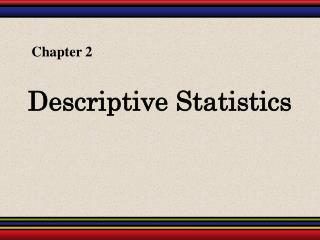DownloadDownload PresentationDescriptive Statistics

# Descriptive Statistics

Télécharger la présentation## Descriptive Statistics

- - - - - - - - - - - - - - - - - - - - - - - - - - - E N D - - - - - - - - - - - - - - - - - - - - - - - - - - -
##### Presentation Transcript

1. Chapter 2 Descriptive Statistics

2. Stem-and-Leaf Plot In a stem-and-leaf plot, each number is separated into a stem (usually the entry’s leftmost digits) and a leaf (usually the rightmost digit). This is an example of exploratory data analysis. Example: The following data represents the ages of 30 students in a statistics class. Display the data in a stem-and-leaf plot. Ages of Students Continued.

3. Most of the values lie between 20 and 39. Stem-and-Leaf Plot Ages of Students Key: 1|8 = 18 8 8 8 9 9 9 1 2 3 4 5 0 0 1 1 1 2 4 7 9 9 0 0 2 2 3 4 7 8 9 4 6 9 1 4 This graph allows us to see the shape of the data as well as the actual values.

4. Stem-and-Leaf Plot Example: Construct a stem-and-leaf plot that has two lines for each stem. Ages of Students Key: 1|8 = 18 1 1 2 2 3 3 4 4 5 5 8 8 8 9 9 9 0 0 1 1 1 2 4 7 9 9 0 0 2 2 3 4 From this graph, we can conclude that more than 50% of the data lie between 20 and 34. 7 8 9 4 6 9 1 4

5. Dot Plot In a dot plot, each data entry is plotted, using a point, above a horizontal axis. Example: Use a dot plot to display the ages of the 30 students in the statistics class. Ages of Students Continued.

6. 15 18 21 24 27 30 33 36 39 42 45 48 51 57 54 Dot Plot Ages of Students From this graph, we can conclude that most of the values lie between 18 and 32.

7. Pie Chart A piechart is a circle that is divided into sectors that represent categories. The area of each sector is proportional to the frequency of each category. Accidental Deaths in the USA in 2002 (Source: US Dept. of Transportation) Continued.

8. Pie Chart To create a pie chart for the data, find the relative frequency (percent) of each category. n = 75,200 Continued.

9. Pie Chart Next, find the central angle. To find the central angle, multiply the relative frequency by 360°. Continued.

10. Pie Chart Firearms 1.9% Ingestion 3.9% Fire 5.6% Drowning 6.1% Poison 8.5% Motor vehicles 57.8° Falls 16.2°

11. Pareto Chart A Pareto chart is a vertical bar graph is which the height of each bar represents the frequency. The bars are placed in order of decreasing height, with the tallest bar to the left. Accidental Deaths in the USA in 2002 (Source: US Dept. of Transportation) Continued.

12. Ingestion of Food/Object Pareto Chart Accidental Deaths 45000 40000 35000 30000 25000 20000 15000 10000 Poison 5000 MotorVehicles Firearms Falls Poison Drowning Fire

13. Scatter Plot When each entry in one data set corresponds to an entry in another data set, the sets are called paired data sets. In a scatter plot, the ordered pairs are graphed as points in a coordinate plane. The scatter plot is used to show the relationship between two quantitative variables. The following scatter plot represents the relationship between the number of absences from a class during the semester and the final grade. Continued.

14. Absences Grade x 8 2 5 12 15 9 6 y 78 92 90 58 43 74 81 Final grade (y) 100 90 80 70 60 50 40 Absences (x) 16 0 2 4 6 8 10 12 14 Scatter Plot From the scatter plot, you can see that as the number of absences increases, the final grade tends to decrease.

15. Times Series Chart A data set that is composed of quantitative data entries taken at regular intervals over a period of time is a timeseries. A timeseries chart is used to graph a time series. Example: The following table lists the number of minutes Robert used on his cell phone for the last six months. Construct a time series chart for the number of minutes used. Continued.

16. Robert’s Cell Phone Usage 250 200 150 Minutes 100 50 0 Jan Feb Mar Apr May June Month Times Series Chart